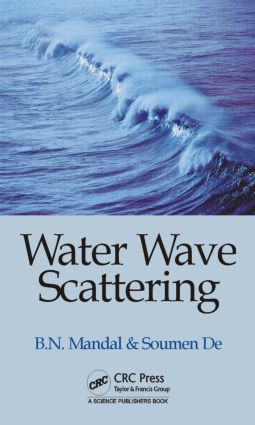Water Wave Scattering

1st Edition

CRC Press

376 pages | 202 B/W Illus.

Hardback: 9781498705523
pub: 2015-05-20
\$155.00
x
eBook (VitalSource) : 9780429067754
pub: 2015-05-21
from \$28.98

FREE Standard Shipping!

Description

The theory of water waves is most varied and is a fascinating topic. It includes a wide range of natural phenomena in oceans, rivers, and lakes. It is mostly concerned with elucidation of some general aspects of wave motion including the prediction of behaviour of waves in the presence of obstacles of some special configurations that are of interest to ocean engineers. Unfortunately, even the apparently simple problems appear to be difficult to tackle mathematically unless some simplified assumptions are made. Fortunately, one can assume water to be an incompressible, in viscid and homogeneous fluid. The linearised theory of water waves is based on the assumption that the amplitude of the motion is small compared to the wave length. If rotational motion is assumed, then the linearised theory of water waves is essentially concerned with solving the Laplace equation in the water region together with linearised boundary condition. There are varied classes of problems that have been/are being studied mathematically in the literature within the framework of linearised theory of water waves for last many years. Scattering by obstacles of various geometrical configurations is one such class of water wave problems. This book is devoted to advanced mathematical work related to water wave scattering. Emphasis is laid on the mathematical and computational techniques required to study these problems mathematically.

The book contains nine chapters. The first chapter is introductory in nature. It includes the basic equations of linearised theory for a single layer fluid, a two-layer fluid, solution of dispersion equations, and a general idea on scattering problems and the energy identity in water with a free surface. Chapter 2 is concerned with wave scattering involving thin rigid plates of various geometrical configurations, namely, plane vertical barriers or curved barriers, inclined barriers, horizontal barrier, and also thin elastic vertical plate. For the horizontal case, the barrier is submerged below an ice-cover modelled as a thin elastic plate floating on water. Chapter 3 discusses wave scattering by a rectangular trench by using Galerkin technique. Chapter 4 involves wave scattering by a dock by using Carleman singular integral equation followed by reduction to Riemann-Hilbert problems. Chapter 5 involves several wave scattering problems involving discontinuities at the upper surface of water by using the Wiener-Hopf technique, by reduction to Carleman singular integral equations. Chapter 6 considers scattering by a long horizontal circular cylinder either half immersed or completely submerged. In chapter 7, some important energy identities are derived for scattering problems in a single-layer and also in a two-layer fluid. Chapter 8 is concerned with wave scattering in a two-layer fluid by a thin vertical plate and by a long horizontal circular cylinder submerged in either of the two layers. Chapter 9 is the final chapter which considers a number of wave scattering problems in a single-layer or a two-layer fluid with variable bottom topography by using a simplified perturbation analysis

It is hoped that this book will be useful to researchers on water waves. The several wave scattering problems presented in the book are mostly based on the research work carried out by the authors and their associates.

Reviews

"The two authors study the linearised theory of water waves. The linearised theory of water waves assumes that the amplitude of the motion is small compared to the wave length. A large number of boundary conditions such as scattering by thin barriers, scattering by rectangular trench and scattering by a semi-in nite dock are investigated. Energy identities are also covered as well as variable bottom topography. In all cases the dispersion relations are discussed in detail. All the details of the calculations are provided. So the authors emphasize the mathematical and computational techniques. An exhaustive reference list is also provided. The book cannot only be recommended for researchers, but also for graduate students."

—Willi-Hans Steeb (Johannesburg), from Zentralblatt MATH 1327 — 1

Introduction

Basic equations of linearised theory water waves

Water with free surface

Water with ice-cover

Higher-order boundary conditions

Solutions of dispersion equations

Scattering problems

Energy identities

Thin barriers

Scattering by two thin vertical barriers

Scattering by two nearly vertical barriers

Scattering by inclined barriers

Scattering by circular arc-shaped thin barrier

Scattering by two circular arc-shaped thin barriers

Scattering by a thin barrier submerged in water with an ice-cover

Scattering by a vertical thin elastic plate

Vertical thick barriers

Scattering by thick rectangular barriers

Scattering by a rectangular trench

Floating dock

Wave scattering by a semi-infinite dock

Surface discontinuities

Scattering by a semi-infinite inertial surface

Solution by Wiener-Hopf Technique

Solution by residue calculus technique

Solution by reducing to singular integral equation

Scattering by a surface strip

Scattering by an elastic strip

Long horizontal cylinder

Scattering by half-immersed circular cylinder

Scattering by submerged cylinder

Two- layer fluid

Scattering by a thin vertical plate

Scattering by a circular cylinder in a two-layer fluid

Scattering by a sphere in a two-layer fluid

Variable bottom topography

Scattering by small deformation of the bottom

Oblique scattering by small bottom deformation

Waves due to a line source in the presence of small bottom deformation

Oblique scattering of interface waves by a small bottom deformation in two superposed fluids

Wave scattering by a small deformation of the bottom of an ocean with an ice-cover

Oblique scattering by undulations on the bed of an ice-covered ocean

Oblique scattering by bottom undulations in a two-layer fluid

Wave scattering by bottom undulations in the presence of a thin partially immersed barrier, a submerged thin vertical plate

Wave propagation over small undulations at the bottom of an ocean with surface discontinuity

Wave scattering by bottom undulations in an ice-covered two-layer fluid